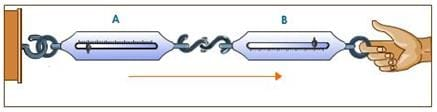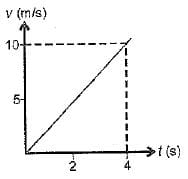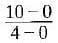UPSC  >  MCQ : Force And Laws Of Motion - 1

# MCQ : Force And Laws Of Motion - 1 - UPSC

Test Description

## 10 Questions MCQ Test Science & Technology for UPSC CSE - MCQ : Force And Laws Of Motion - 1

MCQ : Force And Laws Of Motion - 1 for UPSC 2023 is part of Science & Technology for UPSC CSE preparation. The MCQ : Force And Laws Of Motion - 1 questions and answers have been prepared according to the UPSC exam syllabus.The MCQ : Force And Laws Of Motion - 1 MCQs are made for UPSC 2023 Exam. Find important definitions, questions, notes, meanings, examples, exercises, MCQs and online tests for MCQ : Force And Laws Of Motion - 1 below.
Solutions of MCQ : Force And Laws Of Motion - 1 questions in English are available as part of our Science & Technology for UPSC CSE for UPSC & MCQ : Force And Laws Of Motion - 1 solutions in Hindi for Science & Technology for UPSC CSE course. Download more important topics, notes, lectures and mock test series for UPSC Exam by signing up for free. Attempt MCQ : Force And Laws Of Motion - 1 | 10 questions in 10 minutes | Mock test for UPSC preparation | Free important questions MCQ to study Science & Technology for UPSC CSE for UPSC Exam | Download free PDF with solutions
 1 Crore+ students have signed up on EduRev. Have you?
MCQ : Force And Laws Of Motion - 1 - Question 1

### Newton’s third law of motion explains the two forces namely ‘action’ and ‘reaction’ coming into action when the two bodies are in contact with each other. These two forces:Detailed Solution for MCQ : Force And Laws Of Motion - 1 - Question 1

Always act on the different bodies in opposite directions.

MCQ : Force And Laws Of Motion - 1 - Question 2

### The inertia of an object tends to cause the object ______.

Detailed Solution for MCQ : Force And Laws Of Motion - 1 - Question 2

Inertia of an object is the tendency of the object to resist change in motion. The inertia is directly dependent on the mass of the body.
Inertia resists any change in its state of motion and is of three types:

• Inertia of rest
• Inertia of motion
• Inertia of direction
MCQ : Force And Laws Of Motion - 1 - Question 3

### While catching a stone thrown by your friend you pull the hands back to______.

Detailed Solution for MCQ : Force And Laws Of Motion - 1 - Question 3
• While catching a fast moving cricket ball, a fielder in the ground gradually pulls his hand backward with the moving ball, in doing so, the fielder increases the time during which the high velocity of the moving ball decrease to zero.
• This, the acceleration of the ball is decreased and therefore, the impact of catching the fast moving ball is also reduced.
MCQ : Force And Laws Of Motion - 1 - Question 4

Which one of the following statements is not correct for an object moving along a straight path in an accelerated motion?

Detailed Solution for MCQ : Force And Laws Of Motion - 1 - Question 4
• The question states an accelerated motion of an object along a straight line, this implies a non zero force is acting on the object, which is increasing its velocity and speed.
• However the direction of force is not given so it can’t be determined that it is moving away from the earth, Hence the correct answer is C.
MCQ : Force And Laws Of Motion - 1 - Question 5

The v - t graph of a body of 5 kg moving with the help of a force is shown. Then the force involved is______.Detailed Solution for MCQ : Force And Laws Of Motion - 1 - Question 5

a = slope of graph == 2.5 m/s2
F = ma = 5 x 2.5 = 12.5 N
(c) is correct.

MCQ : Force And Laws Of Motion - 1 - Question 6

Force is defined as:

Detailed Solution for MCQ : Force And Laws Of Motion - 1 - Question 6

"The rate of change of momentum is called force”. “ When a net force acts on a body, it produces acceleration in thr body and will be equal to rate of change of momentum”. Hence , we found that Rate of change of momentum is equal to Force applied on the object .

MCQ : Force And Laws Of Motion - 1 - Question 7

When no external force acts on an object, the physical quantity that remains conserved is______.

Detailed Solution for MCQ : Force And Laws Of Motion - 1 - Question 7

If no external force applied then acceleration will be zero, so velocity remain conserve.
Momentum = mass * velocity, if velocity don't Change momentum also not change.

MCQ : Force And Laws Of Motion - 1 - Question 8

The acceleration of an object is______.

Detailed Solution for MCQ : Force And Laws Of Motion - 1 - Question 8

Newton's second law of motion can be formally stated as follows:- The acceleration of an object as produced by a net force is directly proportional to the magnitude of the net force, in the same direction as the net force, and inversely proportional to the mass of the object.

MCQ : Force And Laws Of Motion - 1 - Question 9

According to second law of Newton, force is the cause and the outcome is______.

Detailed Solution for MCQ : Force And Laws Of Motion - 1 - Question 9

According to Newton’s second law of motion, also known as the Law of Force and Acceleration, a force upon an object's causes it to accelerate according to the formula:
Net force = mass x acceleration.

So, the acceleration of the object is directly proportional to the force and inversely proportional to the mass.

MCQ : Force And Laws Of Motion - 1 - Question 10

Which of the following is an equation of motion of a body?

Detailed Solution for MCQ : Force And Laws Of Motion - 1 - Question 10

v = u +at is the only equation of motion present here in the options. Hence, C is the correct answer

## Science & Technology for UPSC CSE

165 videos|449 docs|327 tests
Information about MCQ : Force And Laws Of Motion - 1 Page
In this test you can find the Exam questions for MCQ : Force And Laws Of Motion - 1 solved & explained in the simplest way possible. Besides giving Questions and answers for MCQ : Force And Laws Of Motion - 1, EduRev gives you an ample number of Online tests for practice

## Science & Technology for UPSC CSE

165 videos|449 docs|327 tests

### How to Prepare for UPSC

Read our guide to prepare for UPSC which is created by Toppers & the best Teachers